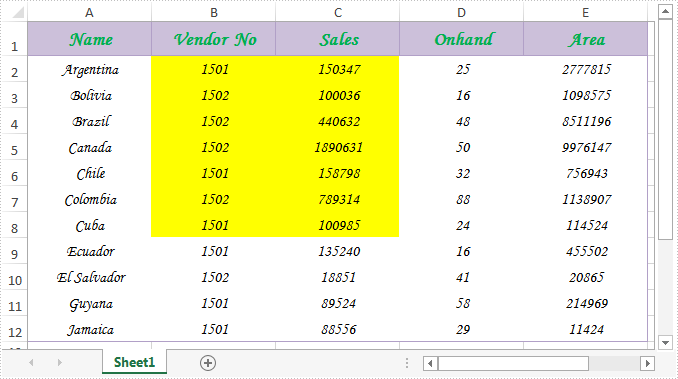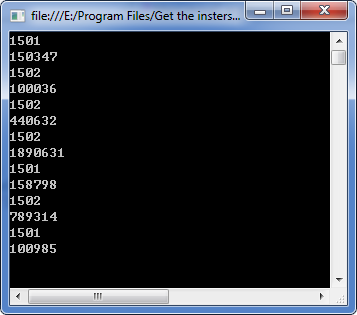# How to get the intersection of two ranges in Excel

Spire.Xls.CellRange class provides a method named Intersect(CellRange range) that is used to find the intersection of certain ranges. This is very useful when we need to get the common value(s) of two ranges in an excel worksheet.

In below picture, we take range A2:C8 and range B2:D8 as an example. Cells filled in yellow color are the intersection of the two ranges.Now refer to the following detail steps:

Step 1: Instantiate an object of Workbook class and load the Excel document.

```Workbook workbook = new Workbook();
```

Step 2: Get the first worksheet.

```Worksheet sheet = workbook.Worksheets;
```

Step 3: Get the intersection of the two ranges and print the common values of them.

```CellRange range = sheet.Range["A2:C8"].Intersect(sheet.Range["B2:D8"]);
foreach (CellRange r in range)
{
Console.WriteLine(r.Value);
}
```

Output:Full code:

[C#]
```using System;
using Spire.Xls;

namespace Get_the_instersection_of_two_ranges
{
class Program
{
static void Main(string[] args)
{
Workbook workbook = new Workbook();
Worksheet sheet = workbook.Worksheets;
CellRange range = sheet.Range["A2:C8"].Intersect(sheet.Range["B2:D8"]);
foreach (CellRange r in range)
{
Console.WriteLine(r.Value);
}
}
}
}
```
[VB.NET]
```Imports Spire.Xls

Namespace Get_the_instersection_of_two_ranges
Class Program
Private Shared Sub Main(args As String())
Dim workbook As New Workbook()